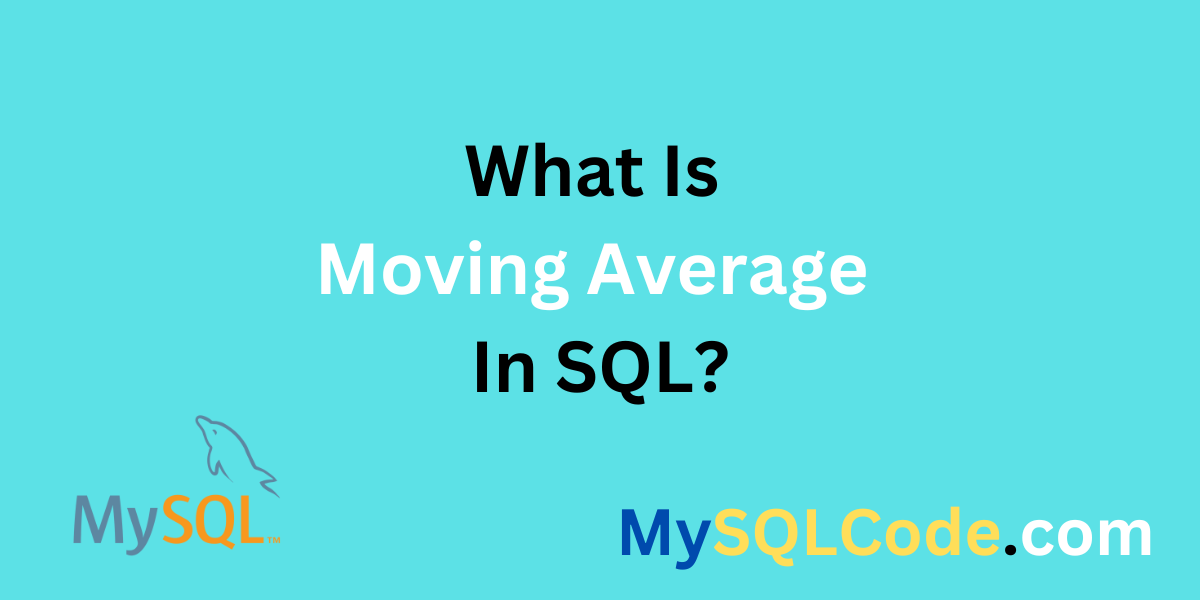# An Introduction to Moving Average in SQLMoving average is related to the time-series databases where we try to calculate an average for all the data points for a particular period. The different databases contain different types of data. Some hold data related to users that visit websites, healthcare data, or some kind of periodic data. This technique is mainly used for stock market databases. Stock market databases have period data where we can apply this model and find out the behaviour. Let’s try to understand the concept of moving average in detail.

## What is the Moving Average?

Moving average is a concept where the average is calculated for periodic data. The moving average is also known as a rolling average or running average. Imagine the data of the stock market where we receive new prizes of stock every time. To analyze this kind of data we need some special technique and that technique is moving average. So, to analyze the trends in time series models, the moving average is used.

## Example of Moving Average In SQL

As we described earlier, the most suitable data to compute the moving average is the stock data. So, let’s try to calculate this moving average with the stock price table. Assume the table has 2 columns: date_of_rec and stock_price. The data is gathered in 10 days. Let’s estimate the moving average of 7 days for this data using SQL query.

Query:

```.wp-block-code {
border: 0;
}

.wp-block-code > span {
display: block;
overflow: auto;
}

.shcb-language {
border: 0;
clip: rect(1px, 1px, 1px, 1px);
-webkit-clip-path: inset(50%);
clip-path: inset(50%);
height: 1px;
margin: -1px;
overflow: hidden;
position: absolute;
width: 1px;
word-wrap: normal;
word-break: normal;
}

.hljs {
box-sizing: border-box;
}

.hljs.shcb-code-table {
display: table;
width: 100%;
}

.hljs.shcb-code-table > .shcb-loc {
color: inherit;
display: table-row;
width: 100%;
}

.hljs.shcb-code-table .shcb-loc > span {
display: table-cell;
}

.wp-block-code code.hljs:not(.shcb-wrap-lines) {
white-space: pre;
}

.wp-block-code code.hljs.shcb-wrap-lines {
white-space: pre-wrap;
}

.hljs.shcb-line-numbers {
border-spacing: 0;
counter-reset: line;
}

.hljs.shcb-line-numbers > .shcb-loc {
counter-increment: line;
}

.hljs.shcb-line-numbers .shcb-loc > span {
}

.hljs.shcb-line-numbers .shcb-loc::before {
border-right: 1px solid #ddd;
content: counter(line);
display: table-cell;
text-align: right;
-webkit-user-select: none;
-moz-user-select: none;
-ms-user-select: none;
user-select: none;
white-space: nowrap;
width: 1%;
}```SELECT
date,
closing_price,
AVG(closing_price) OVER (ORDER BY date ROWS BETWEEN 6 PRECEDING AND CURRENT ROW) AS sma_of_7_day
FROM stock_prices1
ORDER BY date;
```Code language: SQL (Structured Query Language) (sql)```

Output:

In this example, we are calculating the moving average using current data and preceding data using the avg() function and ROWS BETWEEN clause. Therefore, the result should include the recent stock price and moving average of every stock price for 7 days.

This way, we can calculate a moving average for any data using SQL.

## Moving Average vs. Normal Average

The normal average is the usual way of computing an average. It involves counting all the data points and dividing them by the count of data points giving out their normal average. However, a moving average is very complex. A moving average is a type of moving total in which successive input data move to produce a sequence of numbers. From many data points, one can determine a normal average, but that leads to a single result value. The result is also present in a group of values for the moving average.

Normal averages cannot eliminate the noise in the data set. A smoothing mechanism usually involves removing noise from the time-series data which can be carried out through the averaging of sequential points to arrive at a mean value per period. Normally, different averages can apply to any kind of data, but a moving average will be executed in the field of time series data.

## Advantages of Using Moving Average

1. Moving averages help to smooth out data, especially by reducing noise and fluctuations. This helps in highlighting trends and patterns in the data thus making them more identifiable.
2. The volume of data can be reduced without sacrificing relevant information by using moving averages. This comes in handy, especially when handling multiple sets of data.
3. Trends are upward or downward movements that may be detected by moving averages. Such as when a rising moving average implies an upward trend and falling down a moving average signals a downward trend.
4. Sudden steps away from the moving average may indicate abnormalities or unforeseen events concerning the set of data. The same strategy may be applied in looking out for outliers.

## Real-World Applications of Moving Average

1. Moving averages help in revealing seasonal trends and cyclic tendencies of a provided series of information. The use of moving average can be adapted by changing its window size, which enables it to capture other shorter seasonal cycles.
2. Short-term forecasts are possible to visualize using moving average. You can forecast future trends and values by using historical moving averages.
3. Moving averages allow you to make a comparative evaluation of various entities/periods. For instance, consider comparing product categories or region’s moving averages.
4. Moving averages are frequently employed in data visualizations, with continuous lines intended for easy interpretation of time series data.

Working with a database makes it quite easy to calculate moving averages as long as you can use SQL’s built-in functions such as AVG() or window functions.

## Summary

This article is based on the moving average in SQL. The moving average always plays a very important role in analyzing the trends in the field of data analytics. We can directly apply the moving average in SQL. The query uses window functions like AVG() and some other built-in functions. We explained the concept with the stock price database. The real-world applications and advantages of using the moving average in SQL are very wide. The difference between the normal average and the moving average is also explained in detail. Hope you will enjoy this article.

## Reference

https://stackoverflow.com/questions/10624902/sql-moving-average